# TidyTuesday:Video Games

Data Analysis and visualization of the Video Game Data from TidyTuesday week of 2019-07-30.

Diwash Shrestha https://diwashrestha.com.np
08-06-2019

In this post, I will analyse the #Tidytuesday Dataset about a video game from the Steam store.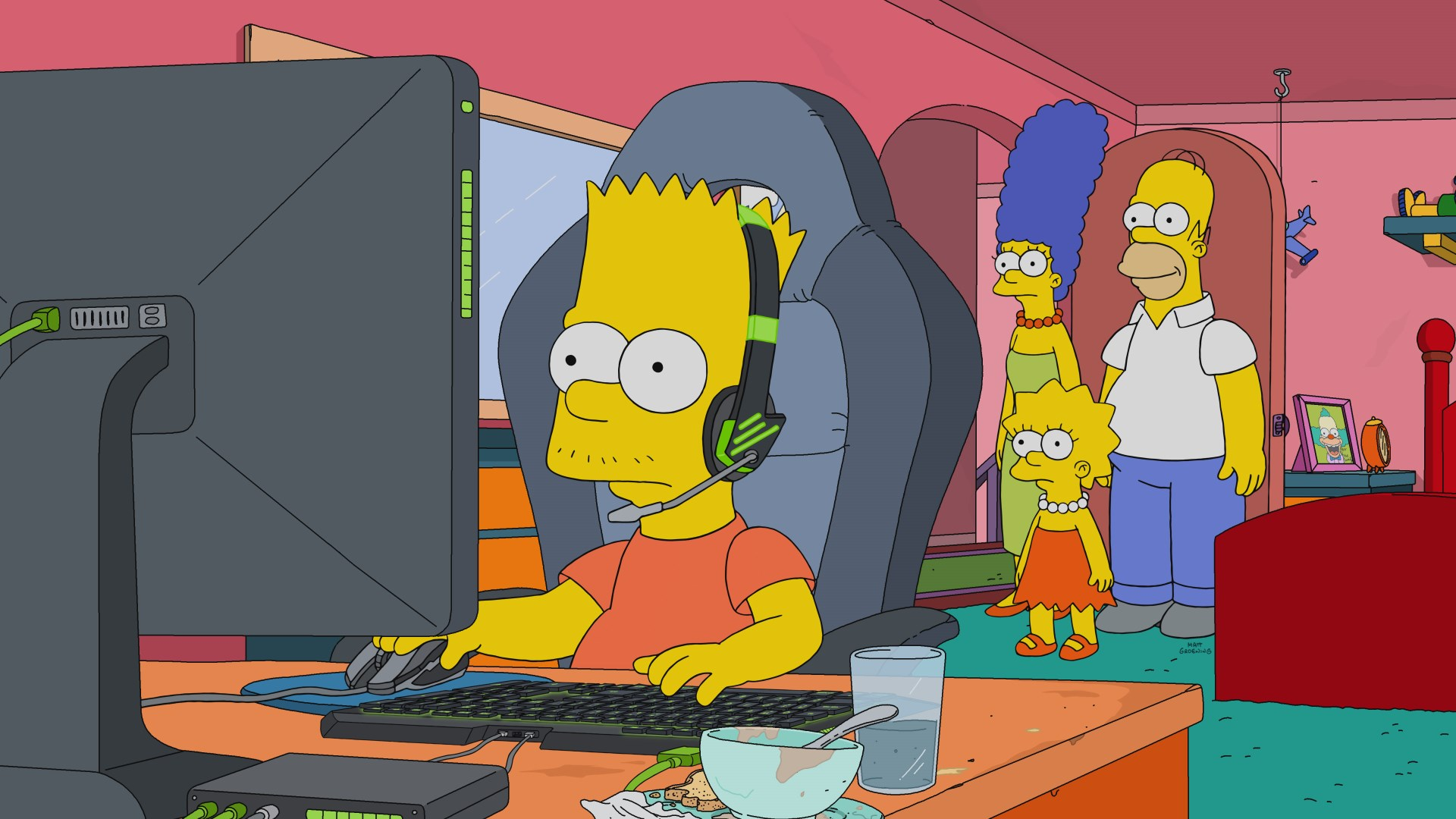PC Gamer

### Load the packages

``````library(tidyverse)
library(lubridate)
library(magrittr)
library(ggthemr)
library(RColorBrewer)
library(gganimate)``````

### Load the data

``````video_game <-
read_csv("https://raw.githubusercontent.com/rfordatascience/tidytuesday/master/data/2019/2019-07-30/video_games.csv")``````

### Data Transformation

Lets take a look at our dataframe.

``head(video_game)``
``````# A tibble: 6 x 10
number game  release_date price owners developer publisher
<dbl> <chr> <chr>        <dbl> <chr>  <chr>     <chr>
1      1 Half~ Nov 16, 2004  9.99 10,00~ Valve     Valve
2      3 Coun~ Nov 1, 2004   9.99 10,00~ Valve     Valve
3     21 Coun~ Mar 1, 2004   9.99 10,00~ Valve     Valve
4     47 Half~ Nov 1, 2004   4.99 5,000~ Valve     Valve
5     36 Half~ Jun 1, 2004   9.99 2,000~ Valve     Valve
6     52 CS2D  Dec 24, 2004 NA    1,000~ Unreal S~ Unreal S~
# ... with 3 more variables: average_playtime <dbl>,
#   median_playtime <dbl>, metascore <dbl>``````
``summary(video_game)``
``````     number         game           release_date
Min.   :   1   Length:26688       Length:26688
1st Qu.: 821   Class :character   Class :character
Median :2356   Mode  :character   Mode  :character
Mean   :2904
3rd Qu.:4523
Max.   :8846

price            owners           developer
Min.   :  0.490   Length:26688       Length:26688
1st Qu.:  2.990   Class :character   Class :character
Median :  5.990   Mode  :character   Mode  :character
Mean   :  8.947
3rd Qu.:  9.990
Max.   :595.990
NA's   :3095
publisher         average_playtime   median_playtime
Length:26688       Min.   :   0.000   Min.   :   0.00
Class :character   1st Qu.:   0.000   1st Qu.:   0.00
Mode  :character   Median :   0.000   Median :   0.00
Mean   :   9.057   Mean   :   5.16
3rd Qu.:   0.000   3rd Qu.:   0.00
Max.   :5670.000   Max.   :3293.00
NA's   :9          NA's   :12
metascore
Min.   :20.00
1st Qu.:66.00
Median :73.00
Mean   :71.89
3rd Qu.:80.00
Max.   :98.00
NA's   :23838  ``````

video_game dataframe has 26688 rows and 10 columns.

### Missing value

Lets check for the missing value in the dataframe.

``colSums(is.na(video_game))``
``````          number             game     release_date            price
0                3                0             3095
owners        developer        publisher average_playtime
0              151               95                9
median_playtime        metascore
12            23838 ``````

Above output shows that We have missing value in this data frame. We will use the median value to replace the missing value of numerical columns.

``````video_game <- video_game %>% mutate(metascore = replace(
metascore,
is.na(metascore),
median(metascore, na.rm = TRUE)
))

video_game <- video_game %>% mutate(price = replace(
price,
is.na(price),
median(price, na.rm = TRUE)
))

video_game <- video_game %>% mutate(average_playtime = replace(
average_playtime,
is.na(average_playtime),
median(average_playtime, na.rm = TRUE)
))

video_game <- video_game %>% mutate(median_playtime = replace(
median_playtime,
is.na(median_playtime),
median(median_playtime, na.rm = TRUE)
))``````
``````video_game <- video_game %>% mutate(
year = year(mdy(release_date)),
month = month(mdy(release_date), label = TRUE),
weekday = wday(mdy(release_date), label = TRUE)
)``````
``video_game <- na.omit(video_game)``
``colSums(is.na(video_game))``
``````          number             game     release_date            price
0                0                0                0
owners        developer        publisher average_playtime
0                0                0                0
median_playtime        metascore             year            month
0                0                0                0
weekday
0 ``````

We replaced the numerical values with the median of the respective values and dropped the missing rows in publisher and developer column.

``````video_game %<>%
mutate(
max_owners = str_trim(word(owners, 2, sep = "\\..")),
max_owners = as.numeric(str_replace_all(max_owners, ",", "")),
min_owners = str_trim(word(owners, 1, sep = "\\..")),
min_owners = as.numeric(str_replace_all(min_owners, ",", ""))
)``````

The owner column has the range of owner of a game and the code create max and min owner of the game.

Let’s see the final data frame:

``head(video_game)``
``````# A tibble: 6 x 15
number game  release_date price owners developer publisher
<dbl> <chr> <chr>        <dbl> <chr>  <chr>     <chr>
1      1 Half~ Nov 16, 2004  9.99 10,00~ Valve     Valve
2      3 Coun~ Nov 1, 2004   9.99 10,00~ Valve     Valve
3     21 Coun~ Mar 1, 2004   9.99 10,00~ Valve     Valve
4      2 Unre~ Mar 16, 2004 15.0  500,0~ Epic Gam~ Epic Gam~
5      4 DOOM~ Aug 3, 2004   4.99 500,0~ id Softw~ id Softw~
6     14 Beyo~ Apr 27, 2004  5.99 500,0~ Larian S~ Larian S~
# ... with 8 more variables: average_playtime <dbl>,
#   median_playtime <dbl>, metascore <dbl>, year <dbl>, month <ord>,
#   weekday <ord>, max_owners <dbl>, min_owners <dbl>``````

### Visualization of Data

``````discrete_pal <- c(
"#fa4234", "#f5b951", "#e8f538", "#52b0f7",
"#b84ef5", "#4ed1f5", "#45b58e", "#1a1612",
"#474ccc", "#47cc92", "#a0cc47", "#c96f32"
)``````
``````ggthemr("flat")
ggplot(data = video_game %>% group_by(developer) %>%
tally(sort = TRUE) %>% head(10)) +
geom_col(aes(x = reorder(developer, n), y = n, fill = n)) +
labs(
title = "Developer with most Games", x = NULL, y = "Game Count",
fill = "Game Count", caption = "by: @diwastha"
) +
theme_minimal() +
theme(axis.text.x = element_text(angle = 40, hjust = 1))``````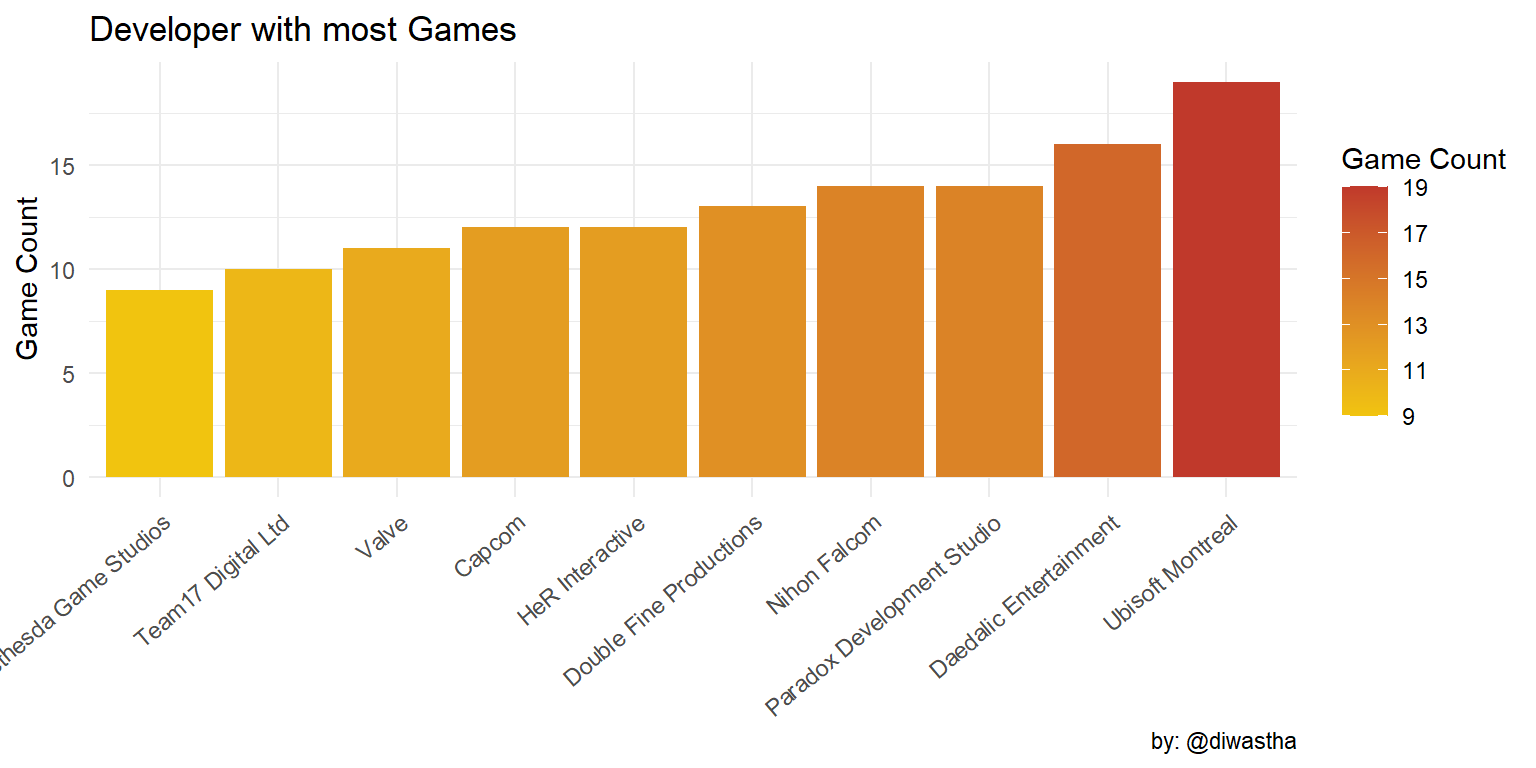``````ggplot(data = video_game %>% group_by(publisher) %>%
tally(sort = TRUE) %>% head(10)) +
geom_col(aes(x = reorder(publisher, n), y = n, fill = n)) +
labs(
title = "Publisher with most Games", y = "Game Count", x = NULL,
fill = "Game Count", caption = "by: @diwastha"
) +
theme_minimal() +
theme(axis.text.x = element_text(angle = 40, hjust = 1))``````Big Fish Games is the biggest publisher by the game count as it publishes lots of free to play and casual games. Sega which is the second-largest publisher has less than half of the game published by the Big Fish Games.

``````video_game %>%
select(-number) %>%
mutate(max_owners = as.factor(max_owners / 1000000)) %>%
group_by(publisher) %>%
mutate(n = n()) %>%
ungroup() %>%
filter(n >= 80) %>%
ggplot(aes(publisher, max_owners, color = publisher)) +
geom_jitter(show.legend = FALSE, size = 2, alpha = 0.5) +
labs(
title = "Distribution of ownership of top publishers",
y = "Estimated Game Ownership per Million", x = NULL
) +
theme_minimal() + scale_color_manual(values = discrete_pal) +
theme(axis.text.x = element_text(angle = 45, hjust = 1)) +
stat_summary(
fun.y = mean, geom = "line", shape = 20,
size = 5, color = "#555555"
)``````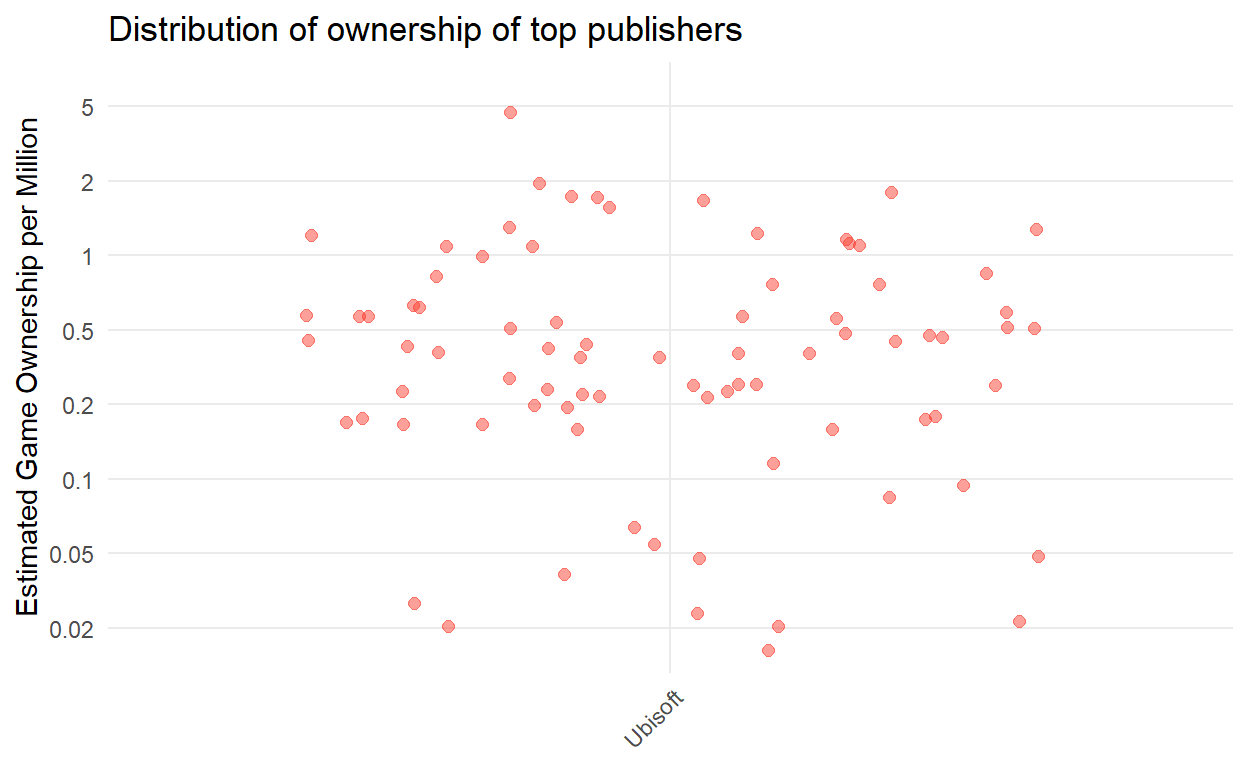The Big Fish Games has released 265 games but the player base of their games is very small. Other publishers in this group have a much larger player base like Ubisoft, Square Enix, SEGA has much larger player base for their games.

``````ggplot(data = video_game %>% arrange(desc(average_playtime)) %>% head(20)) +
geom_col(aes(x = reorder(game, average_playtime), y = average_playtime, fill = metascore)) +
labs(
title = "Games with most metascore", x = NULL, y = "Average Playtime",
caption = "by: @diwastha"
) +
theme_minimal() +
theme(axis.text.x = element_text(angle = 45, hjust = 1))````````````ggplot(data = video_game %>% group_by(max_owners) %>%
arrange(desc(max_owners)) %>% head(10)) +
geom_col(aes(x = reorder(game, max_owners), y = max_owners)) +
labs(
title = "Most Sold Video Games", y = "Number of copies sold", x = NULL,
caption = "by: @diwastha"
) +
theme_minimal() +
theme(axis.text.x = element_text(angle = 45, hjust = 1))``````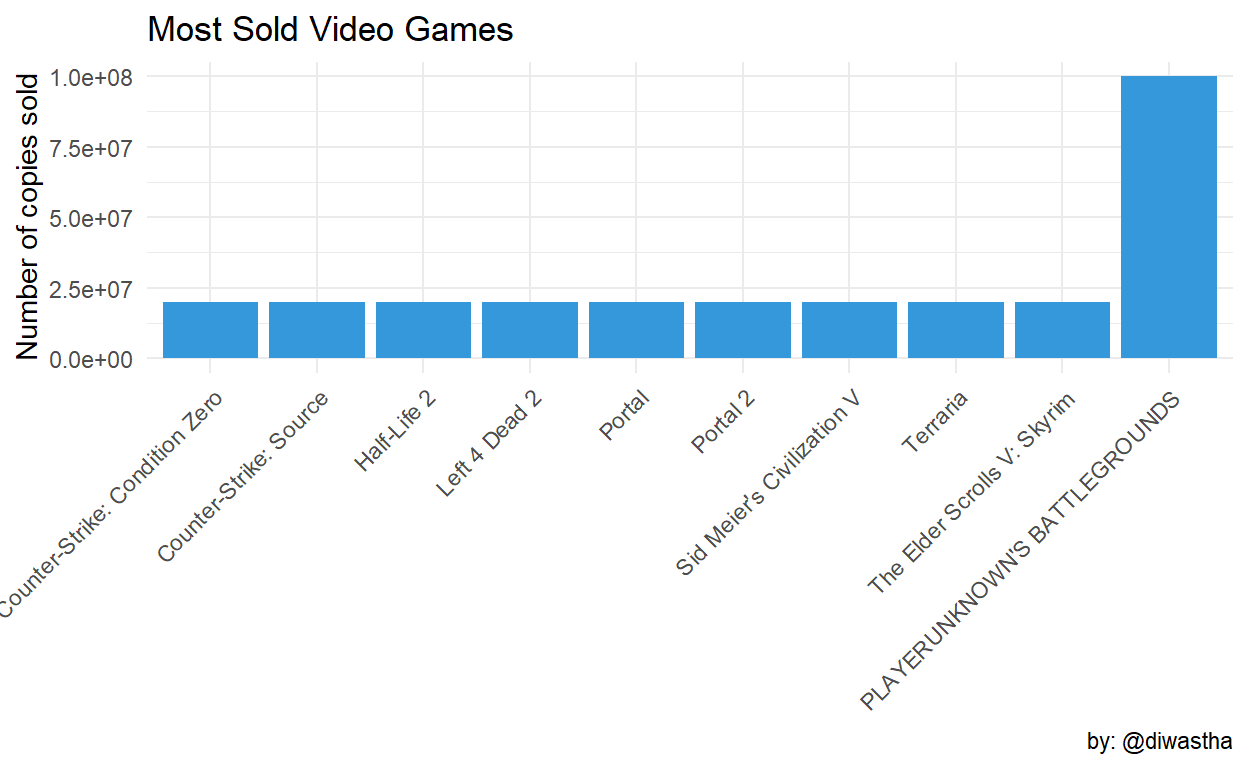Dota2, Team Fortress 2 is free to play multiplayer games released on 2013 and 2007 respectively. The Player Unknown BattleGround(PUBG) is also a multiplayer battle royale shooter which is a huge hit. Counter-Strike is currently available free in steam which may have increased the owner count. All Top five games are multiplayer games with a strong player base and metascore.

``````ggplot(data = video_game %>% group_by(max_owners) %>%
arrange(desc(max_owners)) %>% head(10)) +
geom_col(aes(x = reorder(game, price), y = price, fill = price)) +
labs(
title = "Price of most sold games", y = "Price in \$", x = NULL,
caption = "by: @diwastha"
) +
theme_minimal() +
theme(axis.text.x = element_text(angle = 45, hjust = 1))``````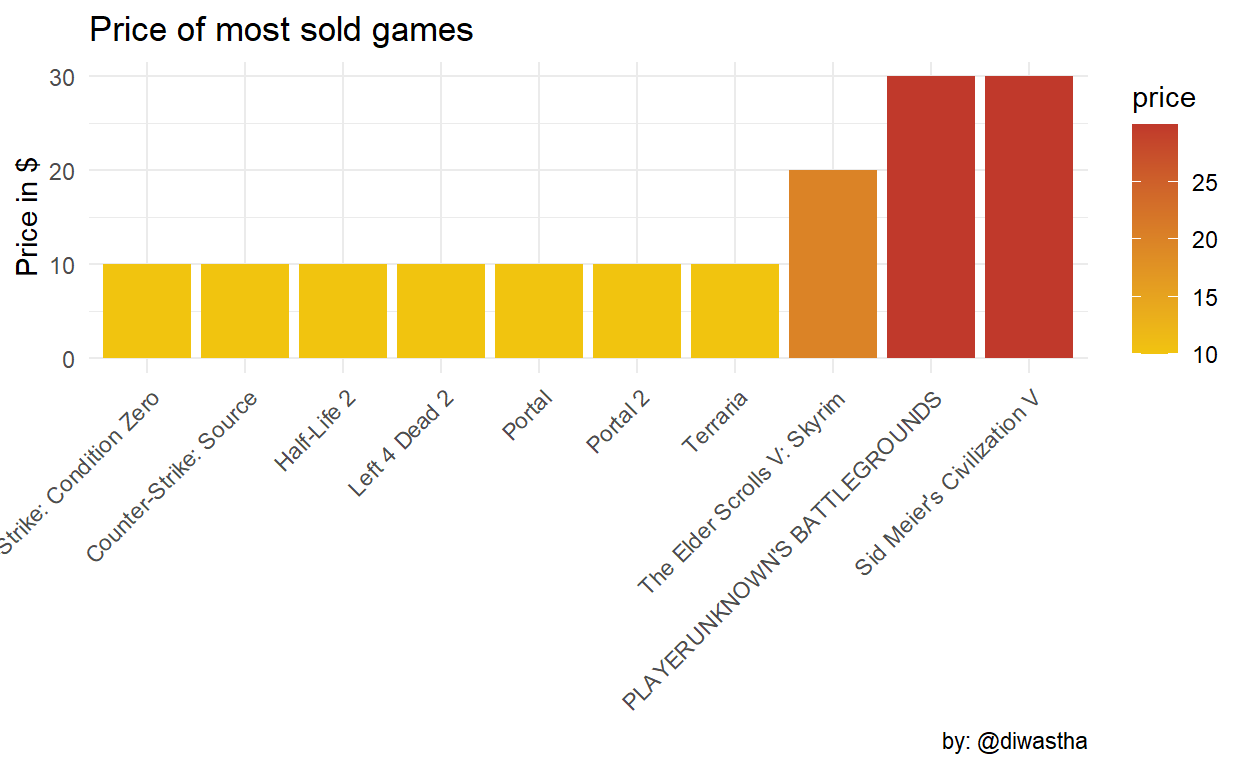Pubg is currently one of the most popular games in the steam store and huge player base. Other games in this group have a very low price or currently free in the steam store.

``````ggthemr("flat")
ggplot(
data = video_game %>% group_by(year) %>%
summarise(avg_price = mean(price)),
aes(x = year, y = avg_price)
) +
geom_line() + geom_point(color = "red") +
labs(
title = "Average Price of the Game over the year",
x = NULL, y = "price in \$", caption = "by: @diwastha"
) +
theme_minimal()``````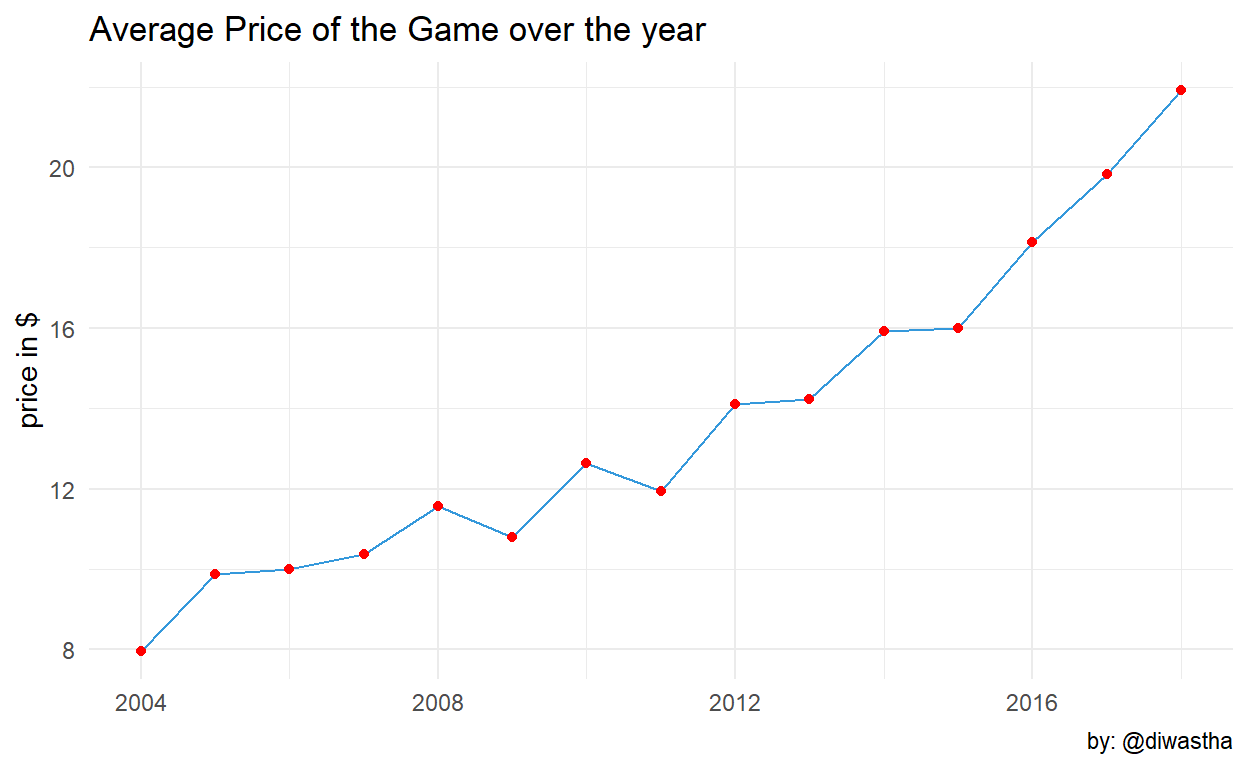The average game price was rising up to the year 2013 then it started to decrease. It may be due to the increment in the release of the game with a very low price or freemium model.

``````ggplot(data = video_game) + geom_bar(aes(x = year)) +
labs(
title = "Number of active games since release year",
y = "Number of Game", x = NULL, caption = "by: @diwastha"
) +
theme_minimal()``````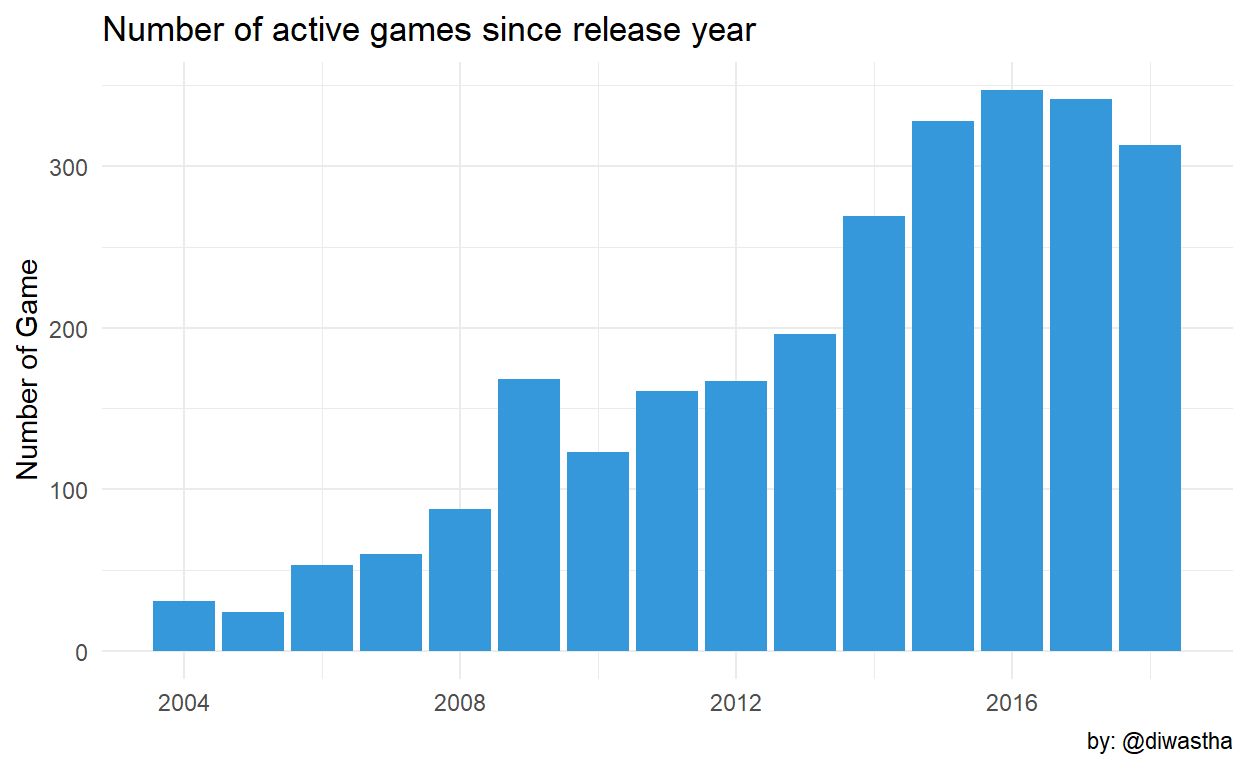There is growth in the release of the games from the year 2015 onward.

``````ggplot(data = video_game) + geom_bar(aes(x = month)) +
transition_time(year) +
labs(
title = "Games released in each month",
subtitle = "Year: {frame_time}",
y = "Number of Game", x = NULL, caption = "by: @diwastha"
) +
theme_minimal()``````The above animation shows the number of games released on each month of the year.

### Wrapping up

Tidytuesday repo has a great dataset for analysis and visualization. You can also extract other data from the repo and work on it. I have taken some references from the work of other people while working on this data and they are:
Anasthsia Kuprina CHRISTOPHER YEE